# The TOP - 25 term deposits for 1 month26.07.2019

## Bankchart.com.au has surveyed the terms of term deposits for 1 month in the national currency. The survey covered all the deposits with any form of interests repayment, regardless of the replenishment option or the deposit partial withdrawal option. Our website's analysts have studied the loan offers from all Australian banks. Review date - 26.07.2019

As of 07 July 2019 the deposits under review have been offered by 25 banks.  The average interest rate under such deposits equals 1,15 % p.a. The total number of the deposits programs available for the specified terms is 49.

The table below shows the best offers by each bank selected by Bankchart.com.au (in terms of profitability) for the aforementioned terms.

### The TOP-25 term deposits for 1 month, as of 07 July 2019

#

Name

Interest Rate, %

1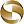Mega International Commercial Bank

«Term Deposits»

1,90

interest rate, %

rate type

fixed

interest periodicity

at maturity

minimum sum

\$ 1 000,00

2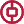Bank of China

«TERM DEPOSIT»

1,90

interest rate, %

rate type

fixed

interest periodicity

at maturity

minimum sum

\$ 500 000,00

3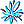AMP Bank

«Term Deposits»

1,65

interest rate, %

rate type

fixed

interest periodicity

at maturity

minimum sum

\$ 500 000,01

4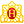Heritage Bank

«Term Deposits»

1,60

interest rate, %

rate type

fixed

interest periodicity

at maturity

minimum sum

\$ 1 000,00

5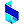Bank of Sydney

«Online Term Deposit»

1,60

interest rate, %

rate type

fixed

interest periodicity

at maturity

minimum sum

\$ 1 000,00

6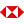HSBC Bank

«Term Deposits»

1,55

interest rate, %

rate type

fixed

interest periodicity

at maturity

minimum sum

\$ 5 000,00

7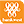Bank of Western Australia

«Online Term Deposit»

1,50

interest rate, %

rate type

fixed

interest periodicity

at maturity, annually

minimum sum

\$ 5 000,00

8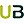Ubank

«Term deposit»

1,50

interest rate, %

rate type

variable

interest periodicity

at maturity

minimum sum

\$ 0,00

9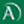Arab Bank

«Fixed Term Deposit»

1,50

interest rate, %

rate type

fixed

interest periodicity

at maturity

minimum sum

\$ 0,00

10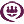RACQ

«Term Deposit»

1,50

interest rate, %

rate type

fixed

interest periodicity

quarterly

minimum sum

\$ 500,00

11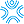Bank First

«Term Deposits»

1,45

interest rate, %

rate type

fixed

interest periodicity

monthly

minimum sum

\$ 500,00

12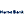Hume Bank

«Term Deposits»

1,40

interest rate, %

rate type

fixed

interest periodicity

at maturity, annually

minimum sum

\$ 5 000,00

13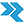Rural Bank

«Short Term Deposit»

1,30

interest rate, %

rate type

fixed

interest periodicity

monthly

minimum sum

\$ 150 000,00

14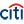Citibank

«Term deposit»

1,28

interest rate, %

rate type

fixed

interest periodicity

monthly

minimum sum

\$ 250 000,00

15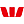Westpac Bank

«Term Deposit»

1,25

interest rate, %

rate type

fixed

interest periodicity

at maturity

minimum sum

\$ 5 000,00

16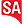Bank SA

«Term Deposits»

1,25

interest rate, %

rate type

fixed

interest periodicity

at maturity

minimum sum

\$ 1 000,00

17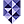Bank of Melbourne

«Term Deposits»

1,25

interest rate, %

rate type

fixed

interest periodicity

at maturity

minimum sum

\$ 1 000,00

18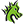St. George Bank

«Term Deposits»

1,25

interest rate, %

rate type

fixed

interest periodicity

at maturity

minimum sum

\$ 1 000,00

19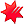National Australia Bank

«NAB Term Deposits»

1,25

interest rate, %

rate type

fixed

interest periodicity

at maturity

minimum sum

\$ 5 000,00

20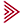Bendigo and Adelaide Bank

«Term deposit»

1,25

interest rate, %

rate type

fixed

interest periodicity

at maturity

minimum sum

\$ 5 000,00

21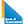Bank of Queensland

«Term deposit»

1,20

interest rate, %

rate type

fixed

interest periodicity

at maturity

minimum sum

\$ 5 000,00

22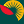Suncorp Bank

«Term Deposit»

1,20

interest rate, %

rate type

fixed

interest periodicity

at maturity

minimum sum

\$ 250 000,00

23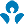Australia and New Zealand (ANZ)

«ANZ Advance Notice Term Deposit interest rates»

1,10

interest rate, %

rate type

fixed

interest periodicity

at maturity

minimum sum

\$ 5 000,00

24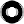Macquarie Bank

«Term Deposit»

1,10

interest rate, %

rate type

fixed

interest periodicity

monthly

minimum sum

\$ 0,00

25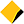Commonwealth Bank of Australia

«Term Deposits»

1,00

interest rate, %

rate type

fixed

interest periodicity

monthly, at maturity, every 6 months

minimum sum

\$ 5 000,00

To view the up-to-date rating of the deposits at our website here.